Goseeko blog# What are State Variables?

The state variables can be explained as a minimal set of states of a system. The knowledge of these variables at any instance of time together with the knowledge of the inputs for the same instance of time, determines the complete behavior of the system. The fewer drawbacks in the transfer function method for representing any system led to the use of state variables in analysis of the system. Few advantages are listed below:

1. In general we use state space in linear or nonlinear, time-variant or time-invariant systems .
2. The places where Laplace transform does not hold good we then implement the state variables.
3. With these we can easily express the nth order differential equation as ‘n’ equation of first order.
4. In time domain system we implement this method.
5. This method is suitable for digital computer computation because it is a time domain method.
6. It can be designed for optimal conditions on the basis of the given performance index.

## Representation of state space:

The system shown below has ‘m’ inputs, ‘p, outputs and ‘n’ number of state variables. Then the state equation gives us the relation between the state variables and the inputs.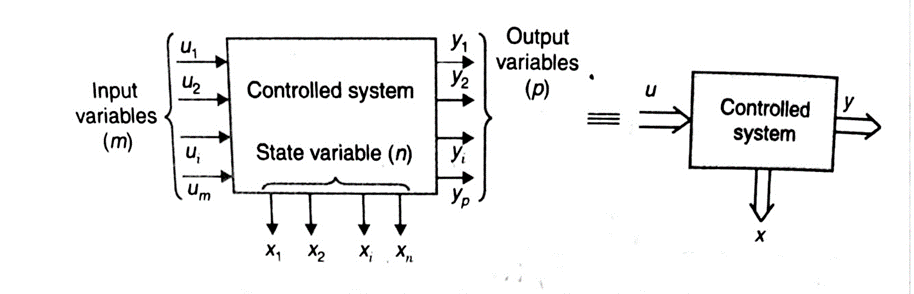So, now the equation for the system above will now beWe can now write the above set of equations as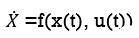As we are concerned for a time invariant system, for which the term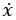is a linear combination of state variables and input. So,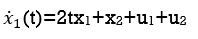Then the matrix form of above equation now becomes as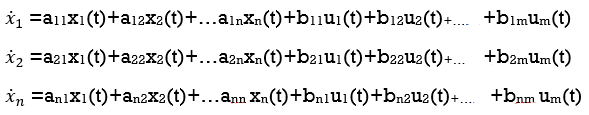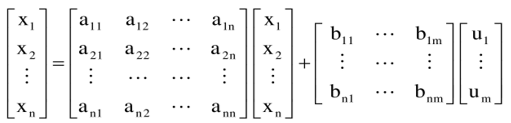The vector matrix form above coefficients aij and bji in equation above will now become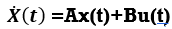Then the linear combination of state variables and inputs represents the output of the system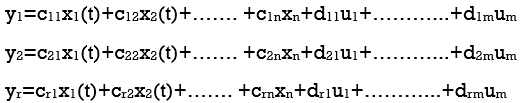The matrix form of equations above in matrix form now becomes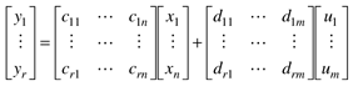Where coefficients cij and dji are constants. Then the equation below is output equation

Y=CX+DU

Interested in learning about similar topics? Here are a few hand-picked blogs for you!